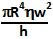## Sunday, June 28, 2009

### Irodov Problem 1.331The viscosity of the fluid causes generates a resistive torque T that tries to slow down the rotating disc. The power generated by by this torque as the disc rotates at an angular velocity of w, is given by Tw.

Now let us compute this resistive torque generated by viscosity. Consider an infinitesimally thin ring of radius r and width dr on the disc. The area of this ring is. Let the resistive viscous force acting on this thin ring be dF.

The velocity of the oil close to this ring section of the disc will be the same as that of the rotating disc equal to wr. The velocity of the oil close to walls of cavity will be 0 since the walls are stationary. Thus, the velocity gradient of the oil in the vertical region between this thin ring section of disc and walls of the cavity will be wr/h.

From the definition of coefficient of viscosity the viscous force dF generated by fluid between the upper cavity wall and the thin ring section of the disc is given by,The same amount of resistive torque is generated by the fluid between the lower wall of the cavity and the thin ring section as well and so the net resistive viscous force is 2(dF). This resistive force 2(dF) results in a resistive torque dT = 2(dF)r given by,The work done by the viscous forces is given by Tw equal to.